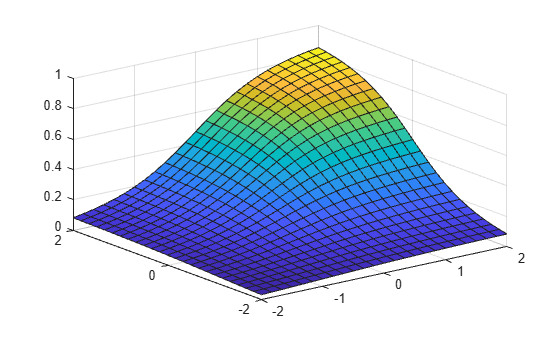# mvtcdf

Multivariate t cumulative distribution function

## Syntax

```y = mvtcdf(X,C,DF) y = mvtcdf(xl,xu,C,DF) [y,err] = mvtcdf(...) [...] = mvntdf(...,options) ```

## Description

`y = mvtcdf(X,C,DF)` returns the cumulative probability of the multivariate t distribution with correlation parameters `C` and degrees of freedom `DF`, evaluated at each row of `X`. Rows of the n-by-d matrix `X` correspond to observations or points, and columns correspond to variables or coordinates. `y` is an `n`-by-`1` vector.

`C` is a symmetric, positive definite, d-by-d matrix, typically a correlation matrix. If its diagonal elements are not 1, `mvtcdf` scales `C` to correlation form. `mvtcdf` does not rescale `X`. `DF` is a scalar, or a vector with n elements.

The multivariate t cumulative probability at `X` is defined as the probability that a random vector `T`, distributed as multivariate t, will fall within the semi-infinite rectangle with upper limits defined by `X`, i.e., `Pr{T(1)``X(1),T(2)``X(2),...T(d)``X(d)}`.

`y = mvtcdf(xl,xu,C,DF)` returns the multivariate t cumulative probability evaluated over the rectangle with lower and upper limits defined by `xl` and `xu`, respectively.

`[y,err] = mvtcdf(...)` returns an estimate of the error in `y`. For bivariate and trivariate distributions, `mvtcdf` uses adaptive quadrature on a transformation of the t density, based on methods developed by Genz, as described in the references. The default absolute error tolerance for these cases is `1e-8`. For four or more dimensions, `mvtcdf` uses a quasi-Monte Carlo integration algorithm based on methods developed by Genz and Bretz, as described in the references. The default absolute error tolerance for these cases is `1e-4`.

`[...] = mvntdf(...,options)` specifies control parameters for the numerical integration used to compute `y`. This argument can be created by a call to `statset`. Choices of `statset` parameters are:

• `'TolFun'` — Maximum absolute error tolerance. Default is `1e-8` when d < 4, or `1e-4` when d ≥ 4.

• `'MaxFunEvals'` — Maximum number of integrand evaluations allowed when d ≥ 4. Default is `1e7`. `'MaxFunEvals'` is ignored when d < 4.

• `'Display'` — Level of display output. Choices are `'off'` (the default), `'iter'`, and `'final'`. `'Display'` is ignored when d < 4.

## Examples

collapse all

Compute the cdf of a multivariate t distribution with correlation parameters `C = [1 .4; .4 1]` and 2 degrees of freedom.

```C = [1 .4; .4 1]; df = 2; [X1,X2] = meshgrid(linspace(-2,2,25)',linspace(-2,2,25)'); X = [X1(:) X2(:)]; p = mvtcdf(X,C,df);```

Plot the cdf.

```figure; surf(X1,X2,reshape(p,25,25));```## References

 Genz, A. “Numerical Computation of Rectangular Bivariate and Trivariate Normal and t Probabilities.” Statistics and Computing. Vol. 14, No. 3, 2004, pp. 251–260.

 Genz, A., and F. Bretz. “Numerical Computation of Multivariate t Probabilities with Application to Power Calculation of Multiple Contrasts.” Journal of Statistical Computation and Simulation. Vol. 63, 1999, pp. 361–378.

 Genz, A., and F. Bretz. “Comparison of Methods for the Computation of Multivariate t Probabilities.” Journal of Computational and Graphical Statistics. Vol. 11, No. 4, 2002, pp. 950–971.